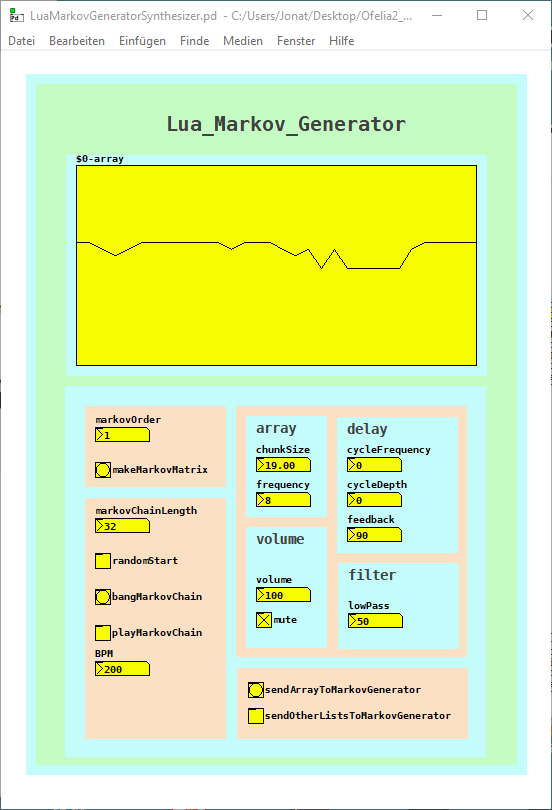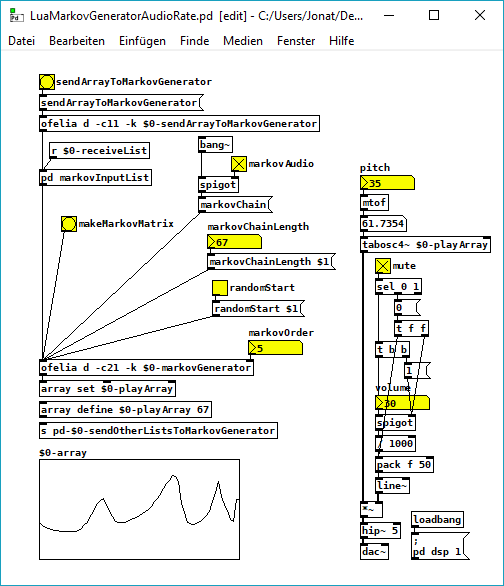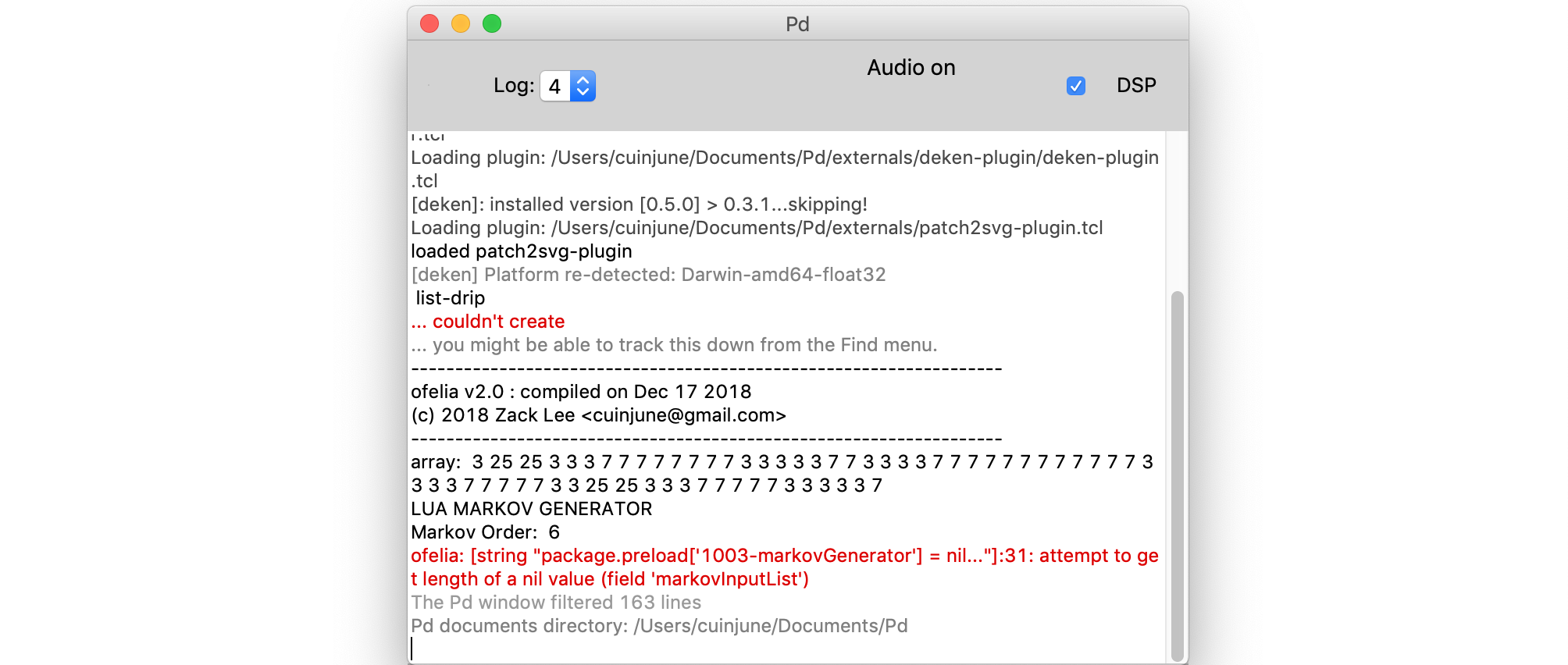• ### Lua / Ofelia Markov Generator Patch / Abstraction

I finished the Ofelia / Lua Markov Generator abstraction / patch.
The markov generator is part of two patches but can easily be used as an abstraction.
I want to use it for pattern variations of a sequencer for example.
It just needs a Pure Data list as input and outputs a markov chain of variable order and length.
Or draw into the array and submit it to the markov generator.
The first patch is an experiment trying to create interesting sounds with the markov algorithm.
In addition I used the variable Delay from the Pure Data help files:
LuaMarkovGeneratorSynthesizer.pdThe second patch creates markov chains at audio rate, it is quite cpu heavy but works until the 10th markov order.
It is quite noisy but I was courius how it will sound:
LuaMarkovGeneratorAudioRate.pdAnd here is the Lua code.
The core of the code is adapted from this python code: https://eli.thegreenplace.net/2018/elegant-python-code-for-a-markov-chain-text-generator/
A few things that I do not really understand yet, but finally it works without errors (it was not easy sometimes):

``````-- LUA MARKOV GENERATOR;
function ofelia.list(fv);
;
math.randomseed(os.time()- os.clock() * 1000);
;
print("LUA MARKOV GENERATOR");
local markovOrder = fv;
print("Markov Order: ", math.floor(markovOrder));
;
-- make dictionary;
;
local function defaultdict(default_value_factory);
;
local t = {};
local metatable = {};
metatable.__index = function(t, key);
if not rawget(t, key) then;
rawset(t, key, default_value_factory(key));
end;
return rawget(t, key);
end;
return setmetatable(t, metatable);
end;
;
-- make markov matrix;
;
local model = defaultdict(function() return {} end);
local data = {};
for i = 1, #ofelia.markovInputList do;
data[i] = ofelia.markovInputList[i];
end;
print("Data Size: ", #ofelia.markovInputList);
for i = 1, markovOrder do;
table.insert(data, data[i]);
end;
for i = 1, #data - markovOrder do;
local state = table.concat({table.unpack(data, i, i + markovOrder - 1)}, "-");
local next = table.unpack(data, i + markovOrder, i + markovOrder);
model[state][next] = (model[state][next] or 0)+1;
end;
;
-- make tables from dict;
;
local keyTbl = {};
local nexTbl = {};
local prbTbl = {};
for key, value in pairs(model) do;
for k, v in pairs(value) do;
table.insert(keyTbl, key);
table.insert(nexTbl, k);
table.insert(prbTbl, v);
end;
end;
;
print("Key: ", table.unpack(keyTbl));
print("Nex: ", table.unpack(nexTbl));
print("Prb: ", table.unpack(prbTbl));
;
print("Make a Markov Chain...");
;
function ofelia.markovChain();
;
-- make start key;
;
local startKey = {};
if ofelia.randomStart == 1 then;
local randomKey = math.random(#keyTbl);
startKey = randomKey;
else;
startKey = 1;
end;
;
local markovString = keyTbl[startKey];
local out = {};
for match in string.gmatch(keyTbl[startKey], "[^-]+") do;
table.insert(out, match);
end;
;
-- make markov chain;
;
for i = 1, ofelia.markovChainLength do;
;
-- weighted random choices;
;
local choices = {};
local weights = {};
for j = 1, #keyTbl do;
if markovString == keyTbl[j] then;
table.insert(choices, nexTbl[j]);
table.insert(weights, prbTbl[j]);
end;
end;
;
-- print ("choices:", table.unpack(choices));
-- print ("weights:", table.unpack(weights));
;
local totalWeight = 0;
for _, weight in pairs(weights) do;
totalWeight = totalWeight + weight;
end;
rand = math.random() * totalWeight;
local choice = nil;
for i, weight in pairs(weights) do;
if rand < weight then;
choice = choices[i];
break;
else;
rand = rand - weight;
end;
end;
;
if math.type(choice) == "integer" then;
choice = choice * (1.0);
end;
;
table.insert(out, choice);
local lastStep = {table.unpack(out, #out - (markovOrder-1), #out)};
markovString = table.concat(lastStep, "-");
end;
;
return {table.unpack(out, markovOrder + 1, #out)};
end;
end;
;
``````
• Posts 5 | Views 600
• @Jona Hi, Thanks for sharing your patch. it looks great!
I just tried your patch but I get the following errors.I get the same errors on Windows too. Do you know how to fix these?

• @Cuinjune Hi. Thanks for trying. The error tells that there is no list in the generator yet. It should be already fixed, it was because of the order creation of Pure Data "Inits". Tell me if not.

• @Jona Hi, I downloaded "list-abs" from Deken and copied "list-drip.pd" into the directory of your patch and now it works fine without the errors.
Thanks!

• @Cuinjune thanks for pointing that out. I overlooked the first error message. I will replace it with the faster list drip with list store from @ingox as a subpatch. https://forum.pdpatchrepo.info/topic/11122/faster-list-drip-with-list-store-pd-0-48

Posts 5 | Views 600
Internal error.

Oops! Looks like something went wrong!